• Shuffle
Toggle On
Toggle Off
• Alphabetize
Toggle On
Toggle Off
• Front First
Toggle On
Toggle Off
• Both Sides
Toggle On
Toggle Off
Toggle On
Toggle Off
Front

## Card Range To Study

throughPlay buttonPlay buttonProgress

1/34

Click to flip

Use LEFT and RIGHT arrow keys to navigate between flashcards;

Use UP and DOWN arrow keys to flip the card;

H to show hint;

 Use objects to represent addition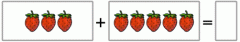Use fingers to represent addition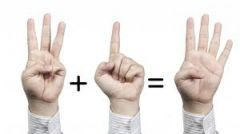Use drawings to represent addition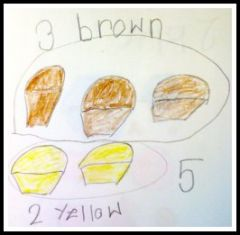Use verbal explanations to represent addition "I started with four apples. I added two more to the group and now there are six apples." Use expressions to represent addition 3+5 2+1 9+1 Use equations to represent addition 3+5=8 2+1=3 9+1=10 Use objects to represent subtraction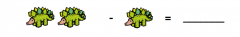Use fingers to represent subtraction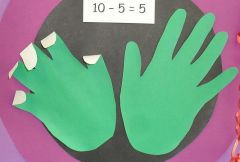Use drawings to represent subtraction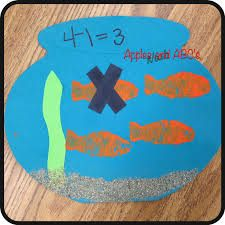Use verbal explanations to represent subtraction "I saw eight birds. Five flew away, so there are three left." Use expressions to represent subtraction 9-1 5-2 6-5 Use equations to represent subtraction 9-1=8 5-2=3 6-5=1 Use objects to solve addition word problems within 10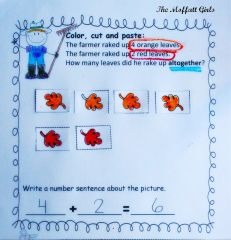Use objects to solve subtraction word problems within 10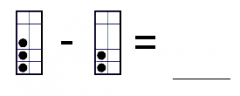My board has 3 circles on it. If I take two circles off my board, how many circles will be left? Use drawings to solve addition word problems within 10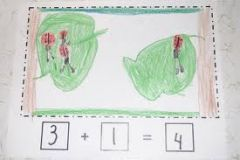There were 3 ladybugs on one leaf, and one ladybug on another leaf. How many ladybugs all together? Use drawings to solve subtraction word problems within 10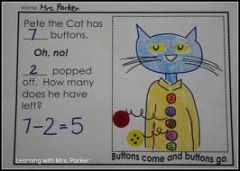Make 10 by adding pairs of numbers 10 = 1+9, 10 = 2+8, 10 = 3+7, 10 = 4+6, 10 = 5+5 Make 9 by adding pairs of numbers 9 = 1+8, 9 = 2+7, 9 = 3+6, 9 = 4+5 Make 8 by adding pairs of numbers 8 = 1+7, 8 = 2+6, 8 = 3+5, 8 = 4+4 Make 7 by adding pairs of numbers 7 = 1+6, 7 = 2+5, 7 = 3+5 Make 6 by adding pairs of numbers 6 = 1+5, 6 = 2+4, 6 = 3+3 Make 5 by adding pairs of numbers 5 = 1+4, 5 = 2+3 Make 4 by adding pairs of numbers 4 = 1+3, 4 = 2+2 Make 3 by adding pairs of numbers 3 = 1+2 Make 2 by adding pairs of numbers 2 = 1+1 What number makes 10 when added to 1? 9 What number makes 10 when added to 2? 8 What number makes 10 when added to 3? 7 What number makes 10 when added to 4? 6 What number makes 10 when added to 5? 5 What number makes 10 when added to 6? 4 What number makes 10 when added to 7? 3 What number makes 10 when added to 8? 2 What number makes 10 when added to 9? 1